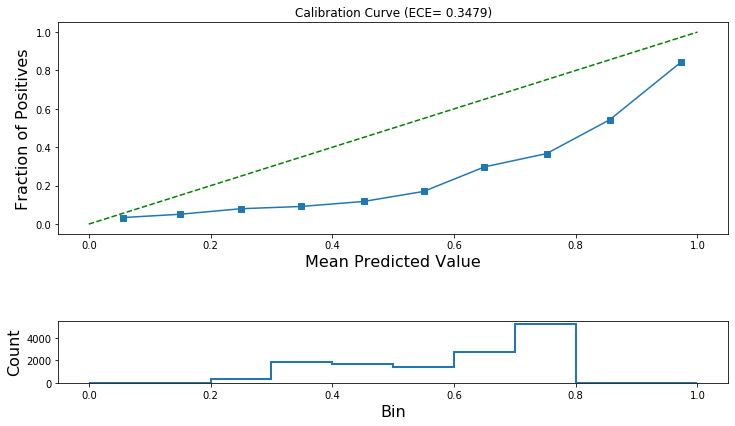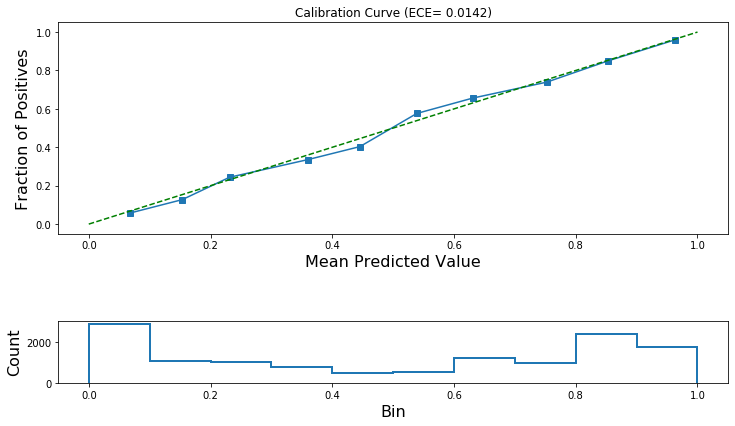Submit a blog to KDnuggets -- Top Blogs Win A Reward

Topics: AI | Data Science | Data Visualization | Deep Learning | Machine Learning | NLP | Python | R | Statistics

KDnuggets Home » News » 2020 » Mar » Tutorials, Overviews » Graph Neural Network model calibration for trusted predictions ( 20:n12 )

# Graph Neural Network model calibration for trusted predictions

In this article, we’ll talk about calibration in graph machine learning, and how it can help to build trust in these powerful new models.

By Pantelis Elinas, CSIRO Data61Graph neural networks (GNNs) are a fast developing machine learning specialisation for classification and regression on graph-structured data. They are a class of powerful representation learning algorithms that map the discrete structure of a graph, e.g., nodes and edges, to a continuous vector representation trainable via stochastic gradient descent.

These representations can be used as input to classification and regression algorithms targeting a variety of applications including finance, genomics, communications, transportation and security. But when applying new machine learning models to real-world problems, we must ask the question: how reliable are they?

In this article, we’ll talk about calibration in graph machine learning, and how it can help to build trust in these powerful new models.

For a detailed overview of graph machine learning and its applications read Knowing your Neighbours: Machine Learning on Graphs

### Classification for graph data

This discussion will focus on only the classification setting for graph data. We consider the problem of predicting a discrete label (binary or multi-class) for the nodes of a graph, given we have observed the labels for a subset of the nodes, their attributes and the graph structure.

In Can Graph Machine Learning Identify Hate Speech in Online Social Networks? we demonstrated the use of a GNN for binary node classification with application in online hate speech detection. In brief, given a network of Twitter users linked via their activity profile, we showed how to use a Graph Convolutional Neural Network (GCN)  to predict if a user was engaging in hateful speech or not.

The GNN model was shown to achieve a higher true positive rate (correctly predicting a higher proportion of hateful users over all known hateful users) for a given false positive rate (incorrectly predicting non-hateful users as hateful) when compared to a traditional machine learning classification model that ignores the graph structure of the data.

Given the above, how much could we trust the GNN model’s prediction if we needed to make a decision on whether to restrict a user’s access to Twitter?

In what follows, we’ll demonstrate how to use and improve the GNN’s predictions to increase our trust of the model and enhance decision making.

### The output of a machine learning classification model

Generally, a machine learning classification model will output, for a given query point, either or both (a) a class label, e.g., hateful or not-hateful, or; (b) a prediction score for each class, e.g., hateful with score 0.8. In the multi-class case, a discrete label for each query point can be obtained by selecting the class assigned the highest predicted score.

In order to train the model and for the label assignment to work consistently across the set of all predicted classes and query points, it is common that the output scores are normalised to lie in the range [0, 1] and sum to one across all classes. That is, we normalise the model’s predicted scores to look like probabilities. For neural network models including GNNs, this normalisation is achieved by adding a softmax output layer.

### Normalised output scores as probabilities

The normalised scores output by the softmax layer have the characteristics of probabilities but do not necessarily share the same semantics. For example, if our GNN predicts that a user is hateful with normalised score 0.7, then if the latter value is interpreted as a probability (as the true posterior probability to be exact), we should find that 70% of similar users are indeed hateful and that 30% are not (hence incorrectly predicted as hateful).

If we use the normalised scores to make probabilistic statements like this, then we should check that the normalised scores output by the model indeed reflect the above proportions. If this is the case then we say that the model predicts well-calibrated probabilities or equivalently that the model is well calibrated.

The advantage of predicting well-calibrated probabilities is that we can be confident in a prediction if the predicted probability is close to 1 or 0, and not so confident if otherwise. For example, if the GNN predicts that a user is hateful with probability 0.9, then we can be confident this prediction is correct for 90% of similar cases considered but only if the probabilities are well-calibrated.

If not, then it is possible that the classifier will over-predict similar users as hateful which may result in the decision to unfairly ban users who are actually not hateful.Photo by Nick Fewings on Unsplash

### Reliability diagrams and Expected Calibration Error (ECE)

Prior studies reveal that some machine learning models predict well-calibrated probabilities while others don’t . For example, popular algorithms such as Support Vector Machines (SVMs) and boosted trees do not predict well-calibrated probabilities. Neural networks do, although it was shown recently that modern deep neural networks are poorly calibrated .

There is also recent work that demonstrates that GNNs are poorly calibrated in some, but not all cases . Given the latter, we should always check if our model is well calibrated. If the model is not well calibrated then we should calibrate it or be cautious about using its prediction to drive decision making.

### How can we determine if our model is well calibrated?

A reliability diagram is commonly used for this purpose, plotting the expected accuracy (fraction of positives) versus prediction confidence (mean predicted value).

To draw such a diagram, we first construct a histogram of the model’s normalised scores using a suitable number of bins, e.g., a 10-bin histogram of the normalised scores that fall in the range [0, 1]. As explained in  and , the fraction of positives is the proportion of points in each bin that belong to the positive class. The mean predicted value for each bin is calculated as the average of the normalised scores assigned to the bin.

Figure 1 below, shows a reliability diagram (calculated using a validation subset of the data, e.g., not used for model training) and the histogram of predicted values for a binary classification problem using a GNN.Figure 1: Reliability diagram for a binary classification model showing the calibration curve (top) and histogram of normalized model predicted scores (bottom). The dashed line indicates the expected calibration curve for a well calibrated model. The solid line is the calibration curve for the trained binary classification GNN model. The GNN model is poorly calibrated as the two lines deviate significantly across the entire range of predicted probabilities.

The reliability diagram for a well-calibrated model will closely follow the diagonal dashed-line shown in the figure. A curve below the diagonal such as the one shown indicates that our model under-predicts the occurrence of the positive class for low true probabilities and over-predicts it for high true probabilities. If a model under-predicts the occurrence of an event across the entire range of true probabilities then the calibration curve is S-shaped.

In some cases, it is preferred that a single numerical value be used to indicate the degree of calibration. One such metric is the Expected Calibration Error (ECE), that is; the weighted average of the difference between expected accuracy and prediction confidence . The difference between these values is calculated for each bin of the histogram used to plot the reliability curve; the weights are simply the proportion of samples that fall in each bin.

The ECE for the example in Figure 1 is approximately 0.35 (35%). A well-calibrated model should have an ECE close to 0.

### Model calibration

Once we have determined that our model is not well calibrated, we have the option of adjusting the model’s predictions using one of several methods. Two commonly used methods are:

• Platt scaling ; and
• Isotonic calibration .

Platt scaling is a parametric calibration method that uses a logistic function to map predicted class scores to well-calibrated probabilities. The input is the values before the softmax layer is applied. The training and/or validation data can be used to estimate the logistic regression model parameters.

Generally, Platt scaling is well suited for poorly calibrated models with an S-shaped calibration curve. Although Platt scaling was originally proposed for calibrating binary classification models, an extension to multi-class classification models called Temperature Scaling was put forward more recently .

Isotonic calibration is a non-parametric method that calculates a non-decreasing function mapping the model’s normalised predicted scores to well-calibrated probabilities. The latter is a key difference compared to Platt scaling since the input to the calibration model are the normalised scores. Isotonic calibration is known to overfit more easily than Platt scaling when data is limited.

We applied isotonic calibration to the uncalibrated model in Figure 1 and then plotted the reliability diagram in Figure 2.Figure 2: Reliability diagram for a binary classification model after the application of Isotonic calibration. Shown are the calibration curve (top) and histogram of calibrated model predicted scores (bottom). The dashed line indicates the expected calibration curve for a well calibrated model. The solid line is the calibration curve for the trained binary classification GNN model after Isotonic calibration.

After calibration, our model predicts well-calibrated probabilities. We see that the reliability curve closely follows the dashed diagonal line. Furthermore, the ECE is now approximately 0.014 (1.4%); that is a considerable reduction compared to the previous value of 0.35 (35%).

### Conclusion

GNN models can produce uncalibrated probabilistic outputs leading to poor decision making and loss of trust. But this problem can be alleviated by the application of a suitable calibration method. We were able to demonstrate that the predicted probabilities of the model shown in Figure 1 could be improved significantly after calibration, as shown in Figure 2.

Stellargraph, an open-source graph machine learning library, implements several state-of-the-art calibration algorithms for GNNs. See this demo Jupyter notebook for an example of calibrating a binary classification model and this demo notebook for an example of calibrating a multi-class classification model.

Given the importance of each decision informed by these models, such as whether to restrict a user’s access to an online social media platform like Twitter, model calibration should be a data scientist’s priority and not an afterthought.

This work is supported by CSIRO’s Data61, Australia’s leading digital research network.### Citations

1. Graph Convolutional Networks (GCN): Semi-Supervised Classification with Graph Convolutional Networks, T. N. Kipf, M. Welling. International Conference on Learning Representations (ICLR), 2017 (link)
2. Predicting Good Probabilities with Supervised Learning, A. Niculescu-Mizil and R. Caruana, ICML 2005 (link)
3. On Calibration of Modern Neural Networks. C. Guo, G. Pleiss, Y. Sun, and K. Q. Weinberger. ICML 2017. (link)
4. Probabilistic Outputs for Support Vector Machines and Comparison to Regularized Likelihood Methods, J. Platt, Advances in Large Margin Classifiers, 1999. (link)
5. Are Graph Neural Networks Miscalibrated?, L. Teixeira, B. Jalaian, and B. Ribeiro, Workshop on Learning and Reasoning with Graph-Structured Representations, ICML 2019. (link)
6. Transforming Classifier Scores into Accurate Multiclass Probability Estimates, B. Zadrozny and C. Elkan, SIGKDD, 2002. (link)

Bio: Pantelis Elinas is a senior machine learning research engineer. He enjoys working on interesting problems, sharing knowledge, and developing useful software tools.

Original. Reposted with permission.

Related:

## Top Stories Past 30 Days

Most PopularGet KDnuggets, a leading newsletter on AI, Data Science, and Machine Learning

By subscribing you accept KDnuggets Privacy Policy